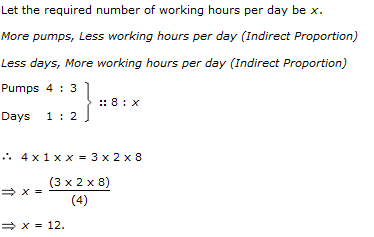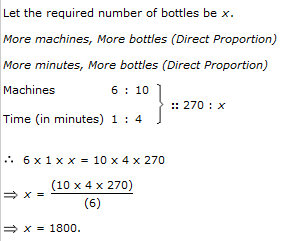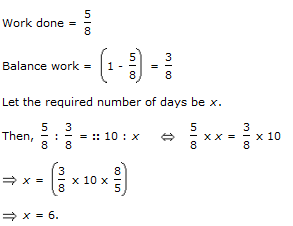1.

3 pumps, working 8 hours a day, can empty a tank in 2 days. How many hours a day must 4 pumps work to empty the tank in 1 day?

 A. 9 B. 10 C. 11 D. 12
Answer: Option D
Explanation:2.

Running at the same constant rate, 6 identical machines can produce a total of 270 bottles per minute. At this rate, how many bottles could 10 such machines produce in 4 minutes?

 A. 648 B. 1800 C. 2700 D. 10800
Answer: Option B
Explanation:3.

A fort had provision of food for 150 men for 45 days. After 10 days, 25 men left the fort. The number of days for which the remaining food will last, is:

 A. 29.5 B. 37.25 C. 42 D. 54
Answer: Option C
Explanation: days : 150 men had food for 35 days.

Suppose 125 men had food for x days.

Now, Less men, More days (Indirect Proportion)

125 : 150 :: 35 : x 125 x x = 150 x 35

x = (150 x 35)/125

x = 42

4.

39 persons can repair a road in 12 days, working 5 hours a day. In how many days will 30 persons, working 6 hours a day, complete the work?

 A. 10 B. 13 C. 14 D. 15
Answer: Option B
Explanation: Let the required number of days be x.

Less persons, More days (Indirect Proportion)

More working hours per day, Less days (Indirect Proportion)

Persons 30 : 39 :: 12 : x

Working hours/day 6 : 5

30 x 6 x x = 39 x 5 x 12

x = (39 x 5 x 12)/(30 x 6)

x = 13

5.

A man completes 5/8 of a job in 10 days. At this rate, how many more days will it takes him to finish the job?

 A. 5 B. 6 C. 7 D. 7.5
Answer: Option B
Explanation: